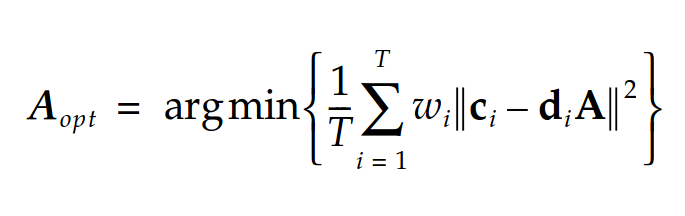# Need help with formulating the following problem using cvx

Hello everybody,

I am quite new to CVX/Matlab optimization and encountering some problems implementing the following expression:where w_i is some weighting factor, c_i is a 1x3 vector of XYZ output values, d_i is a 1x3 vector of RGB input values, A is the 3x3 matrix mapping RGB->XYZ that need to be optimized, and T is the number of the training color sample used for the optimization.

My question is, how can I deal with the summation over indexed variables within the L^2 norm?

Thank you very much for your help!

Perhaps there’s a more elegant way, but you can build up an expression for the objective function in a for loop over i, then minimize it after the loop.

Here’s a general rule. Pretend that you have numeric values of your optimization variable A. How would you compute the numeric value of the objective function in MATLAB?

In many cases, that’s exactly how you would construct the same quantity in CVX.

Thank you very much for your help! Everything finally worked out and I could get the optimization run. Good to know that I can build up the objective function in a separate step before it is called in the minimize() command.

Once again, thank you!